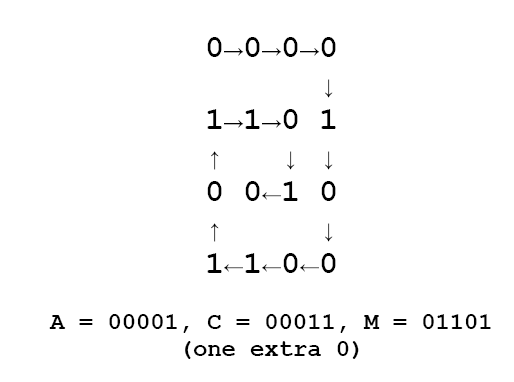# 1935. Decoding

Chip and Dale have devised an encryption method to hide their (written) text messages. They first agree secretly on two numbers that will be used as the number of rows (R) and columns (C) in a matrix. The sender encodes an intermediate format using the following rules:

1. The text is formed with uppercase letters [A-Z] and .

2. Each text character will be represented by decimal values as follows:

= 0, A = 1, B = 2, C = 3, …, Y = 25, Z = 26

The sender enters the 5 digit binary representation of the characters’ values in a spiral pattern along the matrix as shown below. The matrix is padded out with zeroes (0) to fill the matrix completely. For example, if the text to encode is: “ACM” and R=4 and C=4, the matrix would be filled in as follows:The bits in the matrix are then concatenated together in row major order and sent to the receiver. The example above would be encoded as: 0000110100101100

### 输入格式

The first line of input contains a single integer N, (1 ≤ N ≤ 1000) which is the number of datasets that follow.

Each dataset consists of a single line of input containing R (1<=R<=20), a space, C (1<=C<=20), a space, and a string of binary digits that represents the contents of the matrix (R * C binary digits). The binary digits are in row major order.

### 输出格式

For each dataset, you should generate one line of output with the following values: The dataset number as a decimal integer (start counting at one), a space, and the decoded text message. You should throw away any trailing spaces and/or partial characters found while decoding.

### 样例

Input
4
4 4 0000110100101100
5 2 0110000010
2 6 010000001001
5 5 0100001000011010110000010

Output
1 ACM
2 HI
3 HI
4 HI HO


10 人解决，33 人已尝试。

10 份提交通过，共有 139 份提交。

7.8 EMB 奖励。